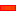﻿ Fixed Gear Centres

# Fixed Gear Centres

## FIXED CENTRES:

 • One Gear-Pair
 • Two or more Gear-Pairs, including
 o Simple Gear-Train
 o Compound Gear-Trains
 o Reverted Gear-Trains

####One Gear-Pair

 Fixed Gear Centres External Gear 1 < Gear 2 External Gear 1 > Gear 2Internal Gear 1 < Gear 2 Internal Gear 1 > Gear 2####Simple Gear-TrainsSchematic of Simple Gear Train

A Simple Gear-Train is a number of Simple Gear-Pairs in series. Each rotating shaft has only one gear.

Simple Gear-Trains are used to:

 • Increase the distance between the input and output shafts, so it is possible to use a number of smaller gears than when you use one gear-pair
 • Reverse the direction of the output shaft of a Simple Gear-Pair

The image to the left shows a schematic of a 'Simple Gear Train'.

Notes for Simple:

 • Gears that are not on the input and output shafts do not change the speed ratio. They only change the direction of rotation.
 • The gears between the input and output shafts are 'idle gears' or 'idlers'.'Monobloc Machines'

This is a schematic of the gears you frequently find on a 'Monobloc' machine. Monobloc are often use for Filling, Capping, Labelling, Coding, Orientating and many other high-speed packaging applications.

Monobloc machines use Simple Gear-Trains to transmit the motion from one 'wheel', or 'head' to the next.

####Compound Gear-TrainsSchematic of Compound, Three-Stage, Gear Train

A 'Compound Gear Train' has at least:

 • two Gear-Pairs
 • one shaft with more than one gear

Compound Gears are used to:

 • Increase the distance between the input and output shafts and still use small gears
 • Dramatically reduce the speed between the input and output shafts
 • Give 'difficult' gear ratios

The image to the left shows a Compound Gear Train. There is more than one gear-pair and two shafts have two gears.

Therefore, it agrees the definition of the Compound Gear Train.

####Reverted Gear-PairsSchematic of Compound, Three-Stage, Gear Train

A 'Reverted Gear Train' has:

 • two Gear-Pairs
 • two shafts
 • the input and output shafts are co-axial
 • the two shafts are usually co-axial

It is a Compound Gear with, two Gears-Pairs, and a special condition that makes the total number of teeth of the first gear-pair equals the number of teeth of the second-gear-pair, when the module is the same.

The image to the left shows a Reverted Gear Train. There is more than one gear-pair, two shafts, and the input and output shafts are collinear.

Therefore, it agrees the definition of the Reverted Gear Train.

Tutorials and Reference Help Files for MechDesigner and MotionDesigner 14.2 + © Machine, Cam, Mechanism, and Motion Design Software by PSMotion Ltd